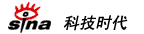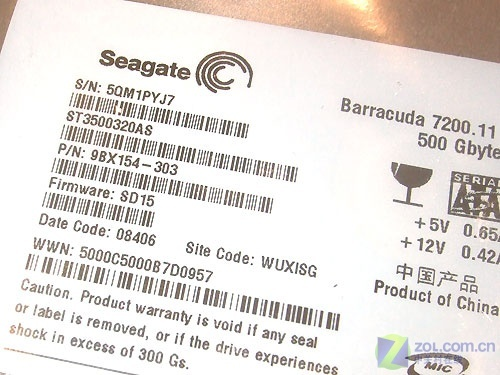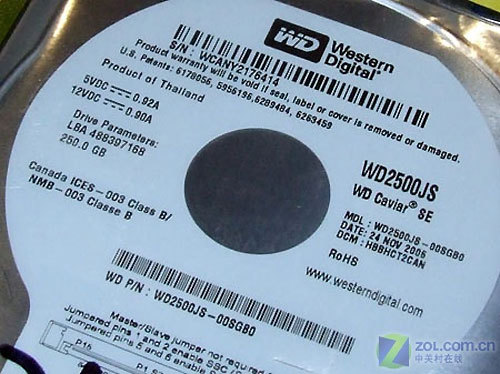.medialogo{float:right; height:21px;} .linkRed01 a{text-decoration:underline;color:#A20010;} .linkRed01 a:hover{color:#f00;}

# 硬件有价数据无价 暑期购买硬盘注意事项(2)

http://www.sina.com.cn 2008年06月30日 06:29  中关村在线

目前市面上销售最好的硬盘主要有希捷、西部数据、日立、三星四个品牌，由于用户喜好不同，笔者下面就对主要品牌的参数进行详细解释，让用户在拿到硬盘时先做到心中有数，以不变应万变。上图为日立500GB 16MB串口台式机硬盘的编号――HDP725050GLA360。下面笔者就将上面的编号拆开，逐一解释。

H = Hitachi（日立标识）
D = Deskstar
P = P7K500系列（S代表Standard）
72 = 7200转
50 = 此系列产品的最大容量为500GB（10=1000GB）
50 = 此款硬盘容量为500GB（16=160GB，25=250GB，32=320GB, 50=500GB, 75=750GB，10=1000GB）
G = 系列代号
L = 标准尺寸
A3 = SATA 3.0Gb/s 接口（AT=PATA 133接口）
6 = 16MB缓存（8=8MB 缓存，6=16MB缓存，3=32MB缓存）
0 = 保留位上图为希捷7200.11 500GB串口硬盘的编号――ST3500320AS。下面笔者就将上面的编号拆开，逐一解释。

ST = Seagate（希捷）
3 = 3.5英寸（1=3.5英寸全高硬盘，3=3.5英寸半高硬盘）
500 = 500GB容量（160=160GB，250=250GB，320=320GB，以此类推）
3 = 32MB缓存（8=8MB，6=16MB）
2 = 两张碟片（1=单碟，3=三碟，4=四碟）
0 = 保留位
S = Serial ATA串行接口（A=PATA并行接口）上图为西部数据250GB 8MB SATA硬盘的编号――WD2500JS，西部数据硬盘编号通常由主编号和附加编号构成。下面笔者就将上面的编号拆开，逐一解释。

WD = Western Digital（西部数据）
2500 = 250GB容量（1600=160GB，5000=500GB，以此类推）
J = 7200rpm/8MB缓存（B=7200转2MB，E=5400转Protege系列，G=10000转8MB）
S = SATA 300MB/s接口（B=ATA接口，D=SATA 150MB/s接口）
00 = 零售市场（非00则是面向OEM客户）
S = 单碟容量
G = 代表同系列硬盘的版本
B0 = 代表硬盘Firmware版本（我们常见的就是A0和B0）

 本文导航: ・硬盘主要参数详解・日立/西数/希捷硬盘编号详解 ・暑期购买硬盘注意事项

 网页 新闻

 ・城市对话改革30年 ・新浪城市同心联动 ・诚招合作伙伴 ・企业邮箱畅通无阻